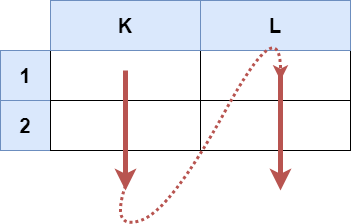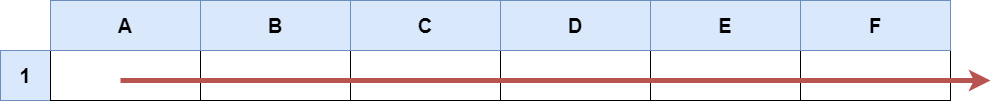| English | 简体中文 |

# 2194. Excel 表中某个范围内的单元格

## 题目描述

Excel 表中的一个单元格 (r, c) 会以字符串 "<col><row>" 的形式进行表示，其中：

• <col> 即单元格的列号 c 。用英文字母表中的 字母 标识。
• 例如，第 1 列用 'A' 表示，第 2 列用 'B' 表示，第 3 列用 'C' 表示，以此类推。
• <row> 即单元格的行号 r 。第 r 行就用 整数 r 标识。输入：s = "K1:L2"输入：s = "A1:F1"



• s.length == 5
• 'A' <= s <= s <= 'Z'
• '1' <= s <= s <= '9'
• s 由大写英文字母、数字、和 ':' 组成